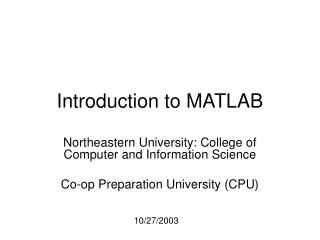# Introduction to MATLAB - PowerPoint PPT PresentationDownload PresentationIntroduction to MATLAB

Introduction to MATLABDownload Presentation## Introduction to MATLAB

- - - - - - - - - - - - - - - - - - - - - - - - - - - E N D - - - - - - - - - - - - - - - - - - - - - - - - - - -
##### Presentation Transcript

1. Introduction to MATLAB Northeastern University: College of Computer and Information Science Co-op Preparation University (CPU) 10/27/2003

2. Overview for 10/27/2003 • Brief review of topics covered in last session (10/23/2003) • Plotting – in depth • File I/O – in depth

3. Review for 10/23/2003 • Functions

4. Sample MATLAB functions • Min • Max • Median • Mean • Sum • Diff

5. MATLAB Functions: Min • Min • Will find the minimum element of the array • Works slightly different on vectors and matrices

6. MATLAB Functions: Max • Max • Will find the maximum element of the array • Also works slightly different on vectors and matrices

7. MATLAB Functions: Median • Median • Will find the median value of the array • Also works slightly different on vectors and matrices

8. MATLAB Functions: Mean • Mean • Returns the average value of the array • Works slightly different on vectors and matrices

9. MATLAB Functions: Sum • Sum • Will return a sum of the array elements • Also works slightly different on vectors and matrices

10. Diff • Diff • Will return the difference between adjacent elements in an array • This is an approximate derivative

11. New MATLAB Function • rand() - Uniformly distributed random numbers and arrays • Ex of syntax: • A = rand(n) • A = rand(m,n) • Where m and n are dimensions of the matrix

12. Rand() con’t: 2 • Scalars may be generated • Ex. A = rand(1,1); • Vectors may be generated • Ex. A = rand(10,1);

13. Rand() con’t: 3 • Generated random numbers will be between 0 and 1. • Scaling can be done by multiplying the resulting matrix or vector by the number you wish to scale with

14. Plotting • Several types of plots available • Plot • Polar • Bar • Hist

15. HAPPYBIRTHDAYWING Here is your pixilated birthday cake. It’s pixa-licious.

16. Plot() (from MATLAB help) • Linear 2-D plot • Syntax: • plot(Y) • plot(X1,Y1,...) • plot(X1,Y1,LineSpec,...) • plot(...,'PropertyName',PropertyValue,...) • h = plot(...)

17. Plot() con’t: 2 • MATLAB defaults to plotting a blue line between points • Other options exist: • Different color lines • Different types of lines • No line at all!

18. Plot() con’t: 3 – Color options • Color options: • Yellow - ‘y’ • Magenta - ‘m’ • Cyan - ‘c’ • Red - ‘r’ • Green - ‘g’ • Blue - ‘b’ • White - ‘w’ • Black - ‘k’ • Example: • plot(temp, ‘y’);

19. Plot() con’t: 4 – Line options • Line styles: • - solid line (default) • -- dashed line • : dotted line • -. dash-dot line

20. Plot() con’t: 5 – Line Markings • + - plus sign • o - circle • * - asterisk • . - Point • x - cross • s - square • d - diamond • ^ - upward pointing triangle • v - downward pointing triangle • > - right pointing triangle • < - left pointing triangle • p - five-pointed star (pentagram) • h - six-pointed star (hexagram)

21. Polar() • Plot polar coordinates • Syntax: • polar(theta,rho) • polar(theta,rho,LineSpec) • Theta – Angle counterclockwise from the 3 o’clock position • Rho – Distance from the origin

22. Polar() con’t: 2 • Line color, style and markings apply as they did in the example with Plot().

23. Bar() • Creates a bar graph • Syntax • bar(Y) • bar(x,Y) • bar(...,width) • bar(...,'style') • bar(...,LineSpec)

24. Hist() • Creates a histogram plot • Syntax: • n = hist(Y) • n = hist(Y,x) • n = hist(Y,nbins)

25. File I/O • Both high-level and low-level file I/O • High-level covered today

26. High-Level File I/O • I/O = input/output • 3 important commands for input • csvread • dlmread • textread